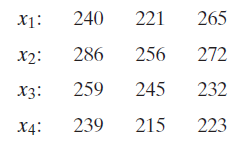×
Get Full Access to Probability And Statistical Inference - 9 Edition - Chapter 9.3 - Problem 9e
Get Full Access to Probability And Statistical Inference - 9 Edition - Chapter 9.3 - Problem 9e

×

# (In some of the exercises that follow, we must | Ch 9.3 - 9EISBN: 9780321923271 41

## Solution for problem 9E Chapter 9.3

Probability and Statistical Inference | 9th Edition

• Textbook Solutions
• 2901 Step-by-step solutions solved by professors and subject experts
• Get 24/7 help from StudySoup virtual teaching assistantsProbability and Statistical Inference | 9th Edition

4 5 1 268 Reviews
13
1
Problem 9E

Let $$X_{i}, i=1,2,3,4$$, equal the distance (in yards) that a golf ball travels when hit from a tee, where $$i$$ denotes the index of the $$i$$ th manufacturer. Assume that the distribution of $$X_{i}$$ is $$N\left(\mu_{i}, \sigma^{2}\right), i=1,2,3,4$$, when a ball is hit by a certain golfer. We shall test the null hypothesis $$H_{0}$$ : $$\mu_{1}=\mu_{2}=\mu_{3}=\mu_{4}$$, using three observations of each random variable.

(a) Give a critical region for an $$\alpha=0.05$$ significance level.

(b) Construct an ANOVA table and state your conclusion, using the following data:(c) What would your conclusion be if $$\alpha=0.025$$ ?

(d) What is the approximate $$p$$-value of this test?

Equation Transcription::Text Transcription:

X_i, i=1,2,3,4

i

X_i

N(mu_i, sigma^2),i=1,2,3,4

H_0: mu_1=mu_2=mu_3=mu_4

alpha=0.05

alpha=0.025

p

Step-by-Step Solution:

Step 1 of 5

Given:

The distance that a golf ball travels when hit from a tee is. It follows normal distribution written as:.

Step 2 of 5

Step 3 of 5

##### ISBN: 9780321923271

The answer to “?Let $$X_{i}, i=1,2,3,4$$, equal the distance (in yards) that a golf ball travels when hit from a tee, where $$i$$ denotes the index of the $$i$$ th manufacturer. Assume that the distribution of $$X_{i}$$ is $$N\left(\mu_{i}, \sigma^{2}\right), i=1,2,3,4$$, when a ball is hit by a certain golfer. We shall test the null hypothesis $$H_{0}$$ : $$\mu_{1}=\mu_{2}=\mu_{3}=\mu_{4}$$, using three observations of each random variable.(a) Give a critical region for an $$\alpha=0.05$$ significance level.(b) Construct an ANOVA table and state your conclusion, using the following data: (c) What would your conclusion be if $$\alpha=0.025$$ ?(d) What is the approximate $$p$$-value of this test?Equation Transcription:: Text Transcription:X_i, i=1,2,3,4 i X_i N(mu_i, sigma^2),i=1,2,3,4 H_0: mu_1=mu_2=mu_3=mu_4 alpha=0.05 alpha=0.025 p” is broken down into a number of easy to follow steps, and 114 words. Since the solution to 9E from 9.3 chapter was answered, more than 361 students have viewed the full step-by-step answer. Probability and Statistical Inference was written by and is associated to the ISBN: 9780321923271. This full solution covers the following key subjects: ball, Using, test, HIT, equal. This expansive textbook survival guide covers 59 chapters, and 1476 solutions. This textbook survival guide was created for the textbook: Probability and Statistical Inference , edition: 9. The full step-by-step solution to problem: 9E from chapter: 9.3 was answered by , our top Statistics solution expert on 07/05/17, 04:50AM.

Unlock Textbook Solution